# 05 - Data Preparation and Advanced Model Evaluation¶

version 1.3, June 2018

# Handling missing values¶

scikit-learn models expect that all values are numeric and hold meaning. Thus, missing values are not allowed by scikit-learn.

In :
import pandas as pd
import zipfile
with zipfile.ZipFile('../datasets/titanic.csv.zip', 'r') as z:
f = z.open('titanic.csv')

Out:
Survived Pclass Name Sex Age SibSp Parch Ticket Fare Cabin Embarked
PassengerId
1 0 3 Braund, Mr. Owen Harris male 22.0 1 0 A/5 21171 7.2500 NaN S
2 1 1 Cumings, Mrs. John Bradley (Florence Briggs Th... female 38.0 1 0 PC 17599 71.2833 C85 C
3 1 3 Heikkinen, Miss. Laina female 26.0 0 0 STON/O2. 3101282 7.9250 NaN S
4 1 1 Futrelle, Mrs. Jacques Heath (Lily May Peel) female 35.0 1 0 113803 53.1000 C123 S
5 0 3 Allen, Mr. William Henry male 35.0 0 0 373450 8.0500 NaN S
In :
# check for missing values
titanic.isnull().sum()

Out:
Survived      0
Pclass        0
Name          0
Sex           0
Age         177
SibSp         0
Parch         0
Ticket        0
Fare          0
Cabin       687
Embarked      2
dtype: int64

One possible strategy is to drop missing values:

In :
# drop rows with any missing values
titanic.dropna().shape

Out:
(183, 11)
In :
# drop rows where Age is missing
titanic[titanic.Age.notnull()].shape

Out:
(714, 11)

Sometimes a better strategy is to impute missing values:

In :
# mean Age
titanic.Age.mean()

Out:
29.69911764705882
In :
# median Age
titanic.Age.median()

Out:
28.0
In :
titanic.loc[titanic.Age.isnull()]

Out:
Survived Pclass Name Sex Age SibSp Parch Ticket Fare Cabin Embarked
PassengerId
6 0 3 Moran, Mr. James male NaN 0 0 330877 8.4583 NaN Q
18 1 2 Williams, Mr. Charles Eugene male NaN 0 0 244373 13.0000 NaN S
20 1 3 Masselmani, Mrs. Fatima female NaN 0 0 2649 7.2250 NaN C
27 0 3 Emir, Mr. Farred Chehab male NaN 0 0 2631 7.2250 NaN C
29 1 3 O'Dwyer, Miss. Ellen "Nellie" female NaN 0 0 330959 7.8792 NaN Q
30 0 3 Todoroff, Mr. Lalio male NaN 0 0 349216 7.8958 NaN S
32 1 1 Spencer, Mrs. William Augustus (Marie Eugenie) female NaN 1 0 PC 17569 146.5208 B78 C
33 1 3 Glynn, Miss. Mary Agatha female NaN 0 0 335677 7.7500 NaN Q
37 1 3 Mamee, Mr. Hanna male NaN 0 0 2677 7.2292 NaN C
43 0 3 Kraeff, Mr. Theodor male NaN 0 0 349253 7.8958 NaN C
46 0 3 Rogers, Mr. William John male NaN 0 0 S.C./A.4. 23567 8.0500 NaN S
47 0 3 Lennon, Mr. Denis male NaN 1 0 370371 15.5000 NaN Q
48 1 3 O'Driscoll, Miss. Bridget female NaN 0 0 14311 7.7500 NaN Q
49 0 3 Samaan, Mr. Youssef male NaN 2 0 2662 21.6792 NaN C
56 1 1 Woolner, Mr. Hugh male NaN 0 0 19947 35.5000 C52 S
65 0 1 Stewart, Mr. Albert A male NaN 0 0 PC 17605 27.7208 NaN C
66 1 3 Moubarek, Master. Gerios male NaN 1 1 2661 15.2458 NaN C
77 0 3 Staneff, Mr. Ivan male NaN 0 0 349208 7.8958 NaN S
78 0 3 Moutal, Mr. Rahamin Haim male NaN 0 0 374746 8.0500 NaN S
83 1 3 McDermott, Miss. Brigdet Delia female NaN 0 0 330932 7.7875 NaN Q
88 0 3 Slocovski, Mr. Selman Francis male NaN 0 0 SOTON/OQ 392086 8.0500 NaN S
96 0 3 Shorney, Mr. Charles Joseph male NaN 0 0 374910 8.0500 NaN S
102 0 3 Petroff, Mr. Pastcho ("Pentcho") male NaN 0 0 349215 7.8958 NaN S
108 1 3 Moss, Mr. Albert Johan male NaN 0 0 312991 7.7750 NaN S
110 1 3 Moran, Miss. Bertha female NaN 1 0 371110 24.1500 NaN Q
122 0 3 Moore, Mr. Leonard Charles male NaN 0 0 A4. 54510 8.0500 NaN S
127 0 3 McMahon, Mr. Martin male NaN 0 0 370372 7.7500 NaN Q
129 1 3 Peter, Miss. Anna female NaN 1 1 2668 22.3583 F E69 C
141 0 3 Boulos, Mrs. Joseph (Sultana) female NaN 0 2 2678 15.2458 NaN C
155 0 3 Olsen, Mr. Ole Martin male NaN 0 0 Fa 265302 7.3125 NaN S
... ... ... ... ... ... ... ... ... ... ... ...
719 0 3 McEvoy, Mr. Michael male NaN 0 0 36568 15.5000 NaN Q
728 1 3 Mannion, Miss. Margareth female NaN 0 0 36866 7.7375 NaN Q
733 0 2 Knight, Mr. Robert J male NaN 0 0 239855 0.0000 NaN S
739 0 3 Ivanoff, Mr. Kanio male NaN 0 0 349201 7.8958 NaN S
740 0 3 Nankoff, Mr. Minko male NaN 0 0 349218 7.8958 NaN S
741 1 1 Hawksford, Mr. Walter James male NaN 0 0 16988 30.0000 D45 S
761 0 3 Garfirth, Mr. John male NaN 0 0 358585 14.5000 NaN S
767 0 1 Brewe, Dr. Arthur Jackson male NaN 0 0 112379 39.6000 NaN C
769 0 3 Moran, Mr. Daniel J male NaN 1 0 371110 24.1500 NaN Q
774 0 3 Elias, Mr. Dibo male NaN 0 0 2674 7.2250 NaN C
777 0 3 Tobin, Mr. Roger male NaN 0 0 383121 7.7500 F38 Q
779 0 3 Kilgannon, Mr. Thomas J male NaN 0 0 36865 7.7375 NaN Q
784 0 3 Johnston, Mr. Andrew G male NaN 1 2 W./C. 6607 23.4500 NaN S
791 0 3 Keane, Mr. Andrew "Andy" male NaN 0 0 12460 7.7500 NaN Q
793 0 3 Sage, Miss. Stella Anna female NaN 8 2 CA. 2343 69.5500 NaN S
794 0 1 Hoyt, Mr. William Fisher male NaN 0 0 PC 17600 30.6958 NaN C
816 0 1 Fry, Mr. Richard male NaN 0 0 112058 0.0000 B102 S
826 0 3 Flynn, Mr. John male NaN 0 0 368323 6.9500 NaN Q
827 0 3 Lam, Mr. Len male NaN 0 0 1601 56.4958 NaN S
829 1 3 McCormack, Mr. Thomas Joseph male NaN 0 0 367228 7.7500 NaN Q
833 0 3 Saad, Mr. Amin male NaN 0 0 2671 7.2292 NaN C
838 0 3 Sirota, Mr. Maurice male NaN 0 0 392092 8.0500 NaN S
840 1 1 Marechal, Mr. Pierre male NaN 0 0 11774 29.7000 C47 C
847 0 3 Sage, Mr. Douglas Bullen male NaN 8 2 CA. 2343 69.5500 NaN S
850 1 1 Goldenberg, Mrs. Samuel L (Edwiga Grabowska) female NaN 1 0 17453 89.1042 C92 C
860 0 3 Razi, Mr. Raihed male NaN 0 0 2629 7.2292 NaN C
864 0 3 Sage, Miss. Dorothy Edith "Dolly" female NaN 8 2 CA. 2343 69.5500 NaN S
869 0 3 van Melkebeke, Mr. Philemon male NaN 0 0 345777 9.5000 NaN S
879 0 3 Laleff, Mr. Kristo male NaN 0 0 349217 7.8958 NaN S
889 0 3 Johnston, Miss. Catherine Helen "Carrie" female NaN 1 2 W./C. 6607 23.4500 NaN S

177 rows × 11 columns

# most frequent Age¶

titanic.Age.mode()

In :
# fill missing values for Age with the median age
titanic.Age.fillna(titanic.Age.median(), inplace=True)


Another strategy would be to build a KNN model just to impute missing values. How would we do that?

If values are missing from a categorical feature, we could treat the missing values as another category. Why might that make sense?

How do we choose between all of these strategies?

# Handling categorical features¶

How do we include a categorical feature in our model?

• Ordered categories: transform them to sensible numeric values (example: small=1, medium=2, large=3)
• Unordered categories: use dummy encoding (0/1)
In :
titanic.head(10)

Out:
Survived Pclass Name Sex Age SibSp Parch Ticket Fare Cabin Embarked
PassengerId
1 0 3 Braund, Mr. Owen Harris male 22.0 1 0 A/5 21171 7.2500 NaN S
2 1 1 Cumings, Mrs. John Bradley (Florence Briggs Th... female 38.0 1 0 PC 17599 71.2833 C85 C
3 1 3 Heikkinen, Miss. Laina female 26.0 0 0 STON/O2. 3101282 7.9250 NaN S
4 1 1 Futrelle, Mrs. Jacques Heath (Lily May Peel) female 35.0 1 0 113803 53.1000 C123 S
5 0 3 Allen, Mr. William Henry male 35.0 0 0 373450 8.0500 NaN S
6 0 3 Moran, Mr. James male 28.0 0 0 330877 8.4583 NaN Q
7 0 1 McCarthy, Mr. Timothy J male 54.0 0 0 17463 51.8625 E46 S
8 0 3 Palsson, Master. Gosta Leonard male 2.0 3 1 349909 21.0750 NaN S
9 1 3 Johnson, Mrs. Oscar W (Elisabeth Vilhelmina Berg) female 27.0 0 2 347742 11.1333 NaN S
10 1 2 Nasser, Mrs. Nicholas (Adele Achem) female 14.0 1 0 237736 30.0708 NaN C
In :
# encode Sex_Female feature
titanic['Sex_Female'] = titanic.Sex.map({'male':0, 'female':1})

In :
# create a DataFrame of dummy variables for Embarked
embarked_dummies = pd.get_dummies(titanic.Embarked, prefix='Embarked')
embarked_dummies.drop(embarked_dummies.columns, axis=1, inplace=True)

# concatenate the original DataFrame and the dummy DataFrame
titanic = pd.concat([titanic, embarked_dummies], axis=1)

In :
titanic.head(1)

Out:
Survived Pclass Name Sex Age SibSp Parch Ticket Fare Cabin Embarked Sex_Female Embarked_Q Embarked_S
PassengerId
1 0 3 Braund, Mr. Owen Harris male 22.0 1 0 A/5 21171 7.25 NaN S 0 0 1
• How do we interpret the encoding for Embarked?
• Why didn't we just encode Embarked using a single feature (C=0, Q=1, S=2)?
• Does it matter which category we choose to define as the baseline?
• Why do we only need two dummy variables for Embarked?
In :
# define X and y
feature_cols = ['Pclass', 'Parch', 'Age', 'Sex_Female', 'Embarked_Q', 'Embarked_S']
X = titanic[feature_cols]
y = titanic.Survived

# train/test split
from sklearn.model_selection import train_test_split
X_train, X_test, y_train, y_test = train_test_split(X, y, random_state=1)

# train a logistic regression model
from sklearn.linear_model import LogisticRegression
logreg = LogisticRegression(C=1e9)
logreg.fit(X_train, y_train)

# make predictions for testing set
y_pred_class = logreg.predict(X_test)

# calculate testing accuracy
from sklearn import metrics
print(metrics.accuracy_score(y_test, y_pred_class))

0.7937219730941704


# ROC curves and AUC¶

In :
# predict probability of survival
y_pred_prob = logreg.predict_proba(X_test)[:, 1]

In :
%matplotlib inline
import matplotlib.pyplot as plt
plt.rcParams['figure.figsize'] = (8, 6)
plt.rcParams['font.size'] = 14

In :
# plot ROC curve
fpr, tpr, thresholds = metrics.roc_curve(y_test, y_pred_prob)
plt.plot(fpr, tpr)
plt.xlim([0.0, 1.0])
plt.ylim([0.0, 1.0])
plt.xlabel('False Positive Rate (1 - Specificity)')
plt.ylabel('True Positive Rate (Sensitivity)')

Out:
Text(0,0.5,'True Positive Rate (Sensitivity)')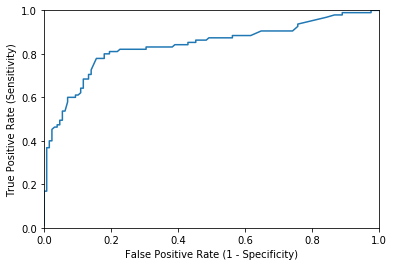In :
# calculate AUC
print(metrics.roc_auc_score(y_test, y_pred_prob))

0.8386924342105262


Besides allowing you to calculate AUC, seeing the ROC curve can help you to choose a threshold that balances sensitivity and specificity in a way that makes sense for the particular context.

In :
# histogram of predicted probabilities grouped by actual response value
df = pd.DataFrame({'probability':y_pred_prob, 'actual':y_test})
df.hist(column='probability', by='actual', sharex=True, sharey=True)

Out:
array([<matplotlib.axes._subplots.AxesSubplot object at 0x7f96c7564160>,
<matplotlib.axes._subplots.AxesSubplot object at 0x7f96c742eac8>],
dtype=object)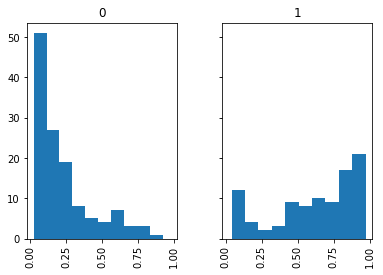In :
# ROC curve using y_pred_class - WRONG!
fpr, tpr, thresholds = metrics.roc_curve(y_test, y_pred_class)
plt.plot(fpr, tpr)

Out:
[<matplotlib.lines.Line2D at 0x7f96c73a7f60>]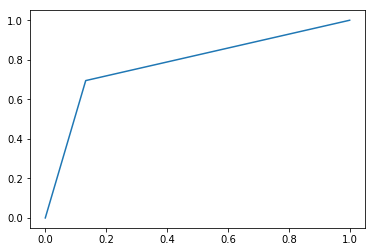In :
# AUC using y_pred_class - WRONG!
print(metrics.roc_auc_score(y_test, y_pred_class))

0.7809621710526315


If you use y_pred_class, it will interpret the zeros and ones as predicted probabilities of 0% and 100%.

# Cross-validation¶

## Review of model evaluation procedures¶

Motivation: Need a way to choose between machine learning models

• Goal is to estimate likely performance of a model on out-of-sample data

Initial idea: Train and test on the same data

• But, maximizing training accuracy rewards overly complex models which overfit the training data

Alternative idea: Train/test split

• Split the dataset into two pieces, so that the model can be trained and tested on different data
• Testing accuracy is a better estimate than training accuracy of out-of-sample performance
• But, it provides a high variance estimate since changing which observations happen to be in the testing set can significantly change testing accuracy
In :
from sklearn.linear_model import LogisticRegression
from sklearn.model_selection import train_test_split
from sklearn import metrics

# define X and y
feature_cols = ['Pclass', 'Parch', 'Age', 'Sex_Female', 'Embarked_Q', 'Embarked_S']
X = titanic[feature_cols]
y = titanic.Survived

In :
# train/test split
X_train, X_test, y_train, y_test = train_test_split(X, y, random_state=1)

# train a logistic regression model
logreg = LogisticRegression(C=1e9)
logreg.fit(X_train, y_train)

# make predictions for testing set
y_pred_class = logreg.predict(X_test)

# calculate testing accuracy
print(metrics.accuracy_score(y_test, y_pred_class))

0.7937219730941704

In :
# train/test split
X_train, X_test, y_train, y_test = train_test_split(X, y, random_state=2)

# train a logistic regression model
logreg = LogisticRegression(C=1e9)
logreg.fit(X_train, y_train)

# make predictions for testing set
y_pred_class = logreg.predict(X_test)

# calculate testing accuracy
print(metrics.accuracy_score(y_test, y_pred_class))

0.7802690582959642

In :
res=[]
for i in range(100):
# train/test split
X_train, X_test, y_train, y_test = train_test_split(X, y, random_state=3*i)

# train a logistic regression model
logreg = LogisticRegression(C=1e9)
logreg.fit(X_train, y_train)

# make predictions for testing set
y_pred_class = logreg.predict(X_test)

# calculate testing accuracy
res.append(metrics.accuracy_score(y_test, y_pred_class))

In :
pd.Series(res).plot()

Out:
<matplotlib.axes._subplots.AxesSubplot at 0x7f96c70eefd0>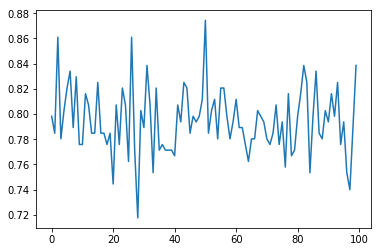train test spliting create bias due to the intrinsic randomness in the sets selection

# K-fold cross-validation¶

1. Split the dataset into K equal partitions (or "folds").
2. Use fold 1 as the testing set and the union of the other folds as the training set.
3. Calculate testing accuracy.
4. Repeat steps 2 and 3 K times, using a different fold as the testing set each time.
5. Use the average testing accuracy as the estimate of out-of-sample accuracy.

Diagram of 5-fold cross-validation: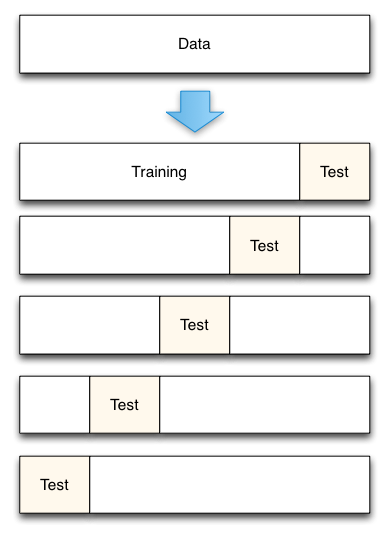In :
# simulate splitting a dataset of 25 observations into 5 folds
from sklearn.cross_validation import KFold
kf = KFold(25, n_folds=5, shuffle=False)

# print the contents of each training and testing set
print('{} {:^61} {}'.format('Iteration', 'Training set observations', 'Testing set observations'))
for iteration, data in enumerate(kf, start=1):
print('{:^9} {} {:^25}'.format(str(iteration), str(data), str(data)))

Iteration                   Training set observations                   Testing set observations
1     [ 5  6  7  8  9 10 11 12 13 14 15 16 17 18 19 20 21 22 23 24]        [0 1 2 3 4]
2     [ 0  1  2  3  4 10 11 12 13 14 15 16 17 18 19 20 21 22 23 24]        [5 6 7 8 9]
3     [ 0  1  2  3  4  5  6  7  8  9 15 16 17 18 19 20 21 22 23 24]     [10 11 12 13 14]
4     [ 0  1  2  3  4  5  6  7  8  9 10 11 12 13 14 20 21 22 23 24]     [15 16 17 18 19]
5     [ 0  1  2  3  4  5  6  7  8  9 10 11 12 13 14 15 16 17 18 19]     [20 21 22 23 24]

• Dataset contains 25 observations (numbered 0 through 24)
• 5-fold cross-validation, thus it runs for 5 iterations
• For each iteration, every observation is either in the training set or the testing set, but not both
• Every observation is in the testing set exactly once
In :
# Create k-folds
kf = KFold(X.shape, n_folds=10, random_state=0)

results = []

for train_index, test_index in kf:
X_train, X_test = X.iloc[train_index], X.iloc[test_index]
y_train, y_test = y.iloc[train_index], y.iloc[test_index]

# train a logistic regression model
logreg = LogisticRegression(C=1e9)
logreg.fit(X_train, y_train)

# make predictions for testing set
y_pred_class = logreg.predict(X_test)

# calculate testing accuracy
results.append(metrics.accuracy_score(y_test, y_pred_class))

In :
pd.Series(results).describe()

Out:
count    10.000000
mean      0.794644
std       0.030631
min       0.764045
25%       0.768820
50%       0.780899
75%       0.820225
max       0.842697
dtype: float64
In :
from sklearn.cross_validation import cross_val_score

logreg = LogisticRegression(C=1e9)

results = cross_val_score(logreg, X, y, cv=10, scoring='accuracy')

In :
pd.Series(results).describe()

Out:
count    10.000000
mean      0.794665
std       0.019263
min       0.775281
25%       0.779963
50%       0.786517
75%       0.806742
max       0.829545
dtype: float64

## Comparing cross-validation to train/test split¶

• More accurate estimate of out-of-sample accuracy
• More "efficient" use of data (every observation is used for both training and testing)

• Runs K times faster than K-fold cross-validation
• Simpler to examine the detailed results of the testing process

## Cross-validation recommendations¶

1. K can be any number, but K=10 is generally recommended
2. For classification problems, stratified sampling is recommended for creating the folds
• Each response class should be represented with equal proportions in each of the K folds
• scikit-learn's cross_val_score function does this by default

## Improvements to cross-validation¶

Repeated cross-validation

• Repeat cross-validation multiple times (with different random splits of the data) and average the results
• More reliable estimate of out-of-sample performance by reducing the variance associated with a single trial of cross-validation

Creating a hold-out set

• "Hold out" a portion of the data before beginning the model building process
• Locate the best model using cross-validation on the remaining data, and test it using the hold-out set
• More reliable estimate of out-of-sample performance since hold-out set is truly out-of-sample

Feature engineering and selection within cross-validation iterations

• Normally, feature engineering and selection occurs before cross-validation
• Instead, perform all feature engineering and selection within each cross-validation iteration
• More reliable estimate of out-of-sample performance since it better mimics the application of the model to out-of-sample data

# Overfitting, Underfitting and Model Selection¶

Now that we've gone over the basics of validation, and cross-validation, it's time to go into even more depth regarding model selection.

The issues associated with validation and cross-validation are some of the most important aspects of the practice of machine learning. Selecting the optimal model for your data is vital, and is a piece of the problem that is not often appreciated by machine learning practitioners.

Of core importance is the following question:

If our estimator is underperforming, how should we move forward?

• Use simpler or more complicated model?
• Add more features to each observed data point?

The answer is often counter-intuitive. In particular, Sometimes using a more complicated model will give worse results. Also, Sometimes adding training data will not improve your results. The ability to determine what steps will improve your model is what separates the successful machine learning practitioners from the unsuccessful.

### Illustration of the Bias-Variance Tradeoff¶

For this section, we'll work with a simple 1D regression problem. This will help us to easily visualize the data and the model, and the results generalize easily to higher-dimensional datasets. We'll explore a simple linear regression problem. This can be accomplished within scikit-learn with the sklearn.linear_model module.

We'll create a simple nonlinear function that we'd like to fit

In :
%matplotlib inline
import numpy as np
import matplotlib.pyplot as plt
def test_func(x, err=0.5):
y = 10 - 1. / (x + 0.1)
if err > 0:
y = np.random.normal(y, err)
return y


Now let's create a realization of this dataset:

In :
def make_data(N=40, error=1.0, random_seed=1):
# randomly sample the data
np.random.seed(1)
X = np.random.random(N)[:, np.newaxis]
y = test_func(X.ravel(), error)

return X, y

In :
X, y = make_data(40, error=1)
plt.scatter(X.ravel(), y);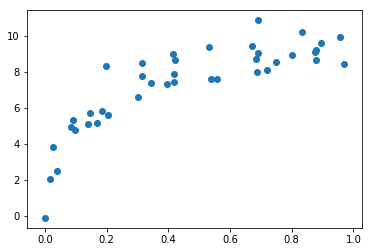Now say we want to perform a regression on this data. Let's use the built-in linear regression function to compute a fit:

In :
X_test = np.linspace(-0.1, 1.1, 500)[:, None]

from sklearn.linear_model import LinearRegression
from sklearn.metrics import mean_squared_error
model = LinearRegression()
model.fit(X, y)
y_test = model.predict(X_test)

plt.scatter(X.ravel(), y)
plt.plot(X_test.ravel(), y_test,c='r')
plt.title("mean squared error: {0:.3g}".format(mean_squared_error(model.predict(X), y)));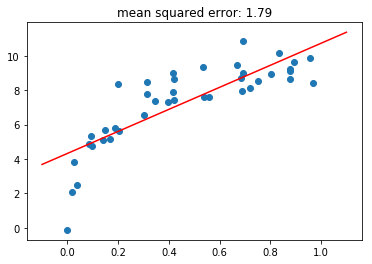We have fit a straight line to the data, but clearly this model is not a good choice. We say that this model is biased, or that it under-fits the data.

Let's try to improve this by creating a more complicated model. We can do this by adding degrees of freedom, and computing a polynomial regression over the inputs. Scikit-learn makes this easy with the PolynomialFeatures preprocessor, which can be pipelined with a linear regression.

Let's make a convenience routine to do this:

In :
from sklearn.preprocessing import PolynomialFeatures
from sklearn.linear_model import LinearRegression


Now we'll use this to fit a quadratic curve to the data.

In :
X_poly = PolynomialFeatures(degree=2).fit_transform(X)
X_test_poly = PolynomialFeatures(degree=2).fit_transform(X_test)

In :
model = LinearRegression()
model.fit(X_poly, y)
y_test = model.predict(X_test_poly)

plt.scatter(X.ravel(), y)
plt.plot(X_test.ravel(), y_test,c='r')
plt.title("mean squared error: {0:.3g}".format(mean_squared_error(model.predict(X_poly), y)));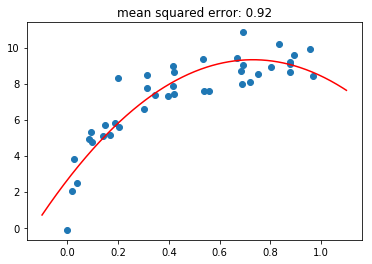This reduces the mean squared error, and makes a much better fit. What happens if we use an even higher-degree polynomial?

In :
X_poly = PolynomialFeatures(degree=30).fit_transform(X)
X_test_poly = PolynomialFeatures(degree=30).fit_transform(X_test)

model = LinearRegression()
model.fit(X_poly, y)
y_test = model.predict(X_test_poly)

plt.scatter(X.ravel(), y)
plt.plot(X_test.ravel(), y_test,c='r')
plt.title("mean squared error: {0:.3g}".format(mean_squared_error(model.predict(X_poly), y)))
plt.ylim(-4, 14);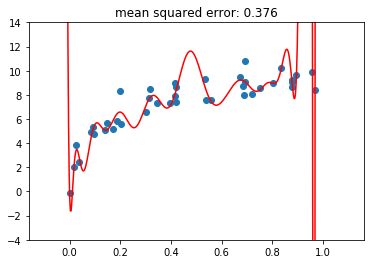When we increase the degree to this extent, it's clear that the resulting fit is no longer reflecting the true underlying distribution, but is more sensitive to the noise in the training data. For this reason, we call it a high-variance model, and we say that it over-fits the data.

### Detecting Over-fitting with Validation Curves¶

Clearly, computing the error on the training data is not enough (we saw this previously). As above, we can use cross-validation to get a better handle on how the model fit is working.

Let's do this here, again using the validation_curve utility. To make things more clear, we'll use a slightly larger dataset:

In :
X, y = make_data(120, error=1.0)
plt.scatter(X, y);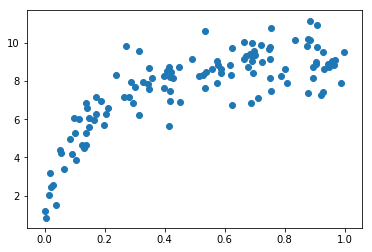In :
from sklearn.model_selection import validation_curve

In :
def rms_error(model, X, y):
y_pred = model.predict(X)
return np.sqrt(np.mean((y - y_pred) ** 2))

In :
from sklearn.pipeline import make_pipeline

def PolynomialRegression(degree=2, **kwargs):
return make_pipeline(PolynomialFeatures(degree),
LinearRegression(**kwargs))

In :
degree = np.arange(0, 18)
val_train, val_test = validation_curve(PolynomialRegression(), X, y,
'polynomialfeatures__degree', degree, cv=7,
scoring=rms_error)


Now let's plot the validation curves:

In :
def plot_with_err(x, data, **kwargs):
mu, std = data.mean(1), data.std(1)
lines = plt.plot(x, mu, '-', **kwargs)
plt.fill_between(x, mu - std, mu + std, edgecolor='none',
facecolor=lines.get_color(), alpha=0.2)

plot_with_err(degree, val_train, label='training scores')
plot_with_err(degree, val_test, label='validation scores')
plt.xlabel('degree'); plt.ylabel('rms error')
plt.legend();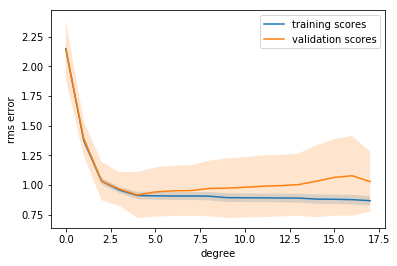Notice the trend here, which is common for this type of plot.

1. For a small model complexity, the training error and validation error are very similar. This indicates that the model is under-fitting the data: it doesn't have enough complexity to represent the data. Another way of putting it is that this is a high-bias model.

2. As the model complexity grows, the training and validation scores diverge. This indicates that the model is over-fitting the data: it has so much flexibility, that it fits the noise rather than the underlying trend. Another way of putting it is that this is a high-variance model.

3. Note that the training score (nearly) always improves with model complexity. This is because a more complicated model can fit the noise better, so the model improves. The validation data generally has a sweet spot, which here is around 5 terms.

Here's our best-fit model according to the cross-validation:

In :
model = PolynomialRegression(4).fit(X, y)
plt.scatter(X, y)
plt.plot(X_test, model.predict(X_test),c='r');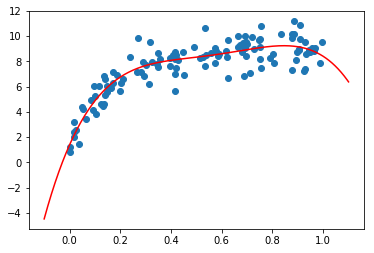### Detecting Data Sufficiency with Learning Curves¶

As you might guess, the exact turning-point of the tradeoff between bias and variance is highly dependent on the number of training points used. Here we'll illustrate the use of learning curves, which display this property.

The idea is to plot the mean-squared-error for the training and test set as a function of Number of Training Points

In :
from sklearn.learning_curve import learning_curve

def plot_learning_curve(degree=3):
train_sizes = np.linspace(0.05, 1, 20)
N_train, val_train, val_test = learning_curve(PolynomialRegression(degree),
X, y, train_sizes, cv=5,
scoring=rms_error)
plot_with_err(N_train, val_train, label='training scores')
plot_with_err(N_train, val_test, label='validation scores')
plt.xlabel('Training Set Size'); plt.ylabel('rms error')
plt.ylim(0, 3)
plt.xlim(5, 80)
plt.legend()

/home/al/anaconda3/lib/python3.6/site-packages/sklearn/learning_curve.py:22: DeprecationWarning: This module was deprecated in version 0.18 in favor of the model_selection module into which all the functions are moved. This module will be removed in 0.20
DeprecationWarning)


Let's see what the learning curves look like for a linear model:

In :
plot_learning_curve(1)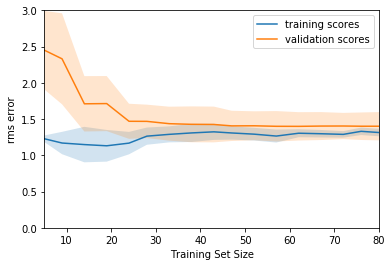This shows a typical learning curve: for very few training points, there is a large separation between the training and test error, which indicates over-fitting. Given the same model, for a large number of training points, the training and testing errors converge, which indicates potential under-fitting.

As you add more data points, the training error will never increase, and the testing error will never decrease (why do you think this is?)

It is easy to see that, in this plot, if you'd like to reduce the MSE down to the nominal value of 1.0 (which is the magnitude of the scatter we put in when constructing the data), then adding more samples will never get you there. For $d=1$, the two curves have converged and cannot move lower. What about for a larger value of $d$?

In :
plot_learning_curve(3)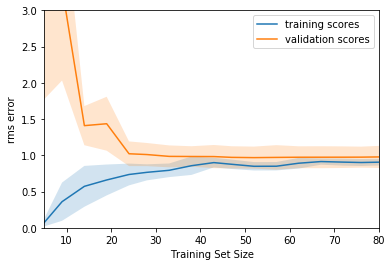Here we see that by adding more model complexity, we've managed to lower the level of convergence to an rms error of 1.0!

What if we get even more complex?

In :
plot_learning_curve(10)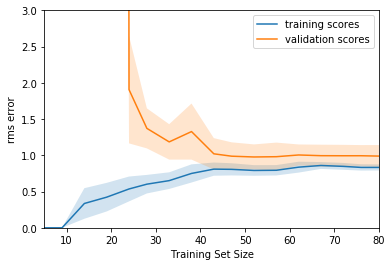For an even more complex model, we still converge, but the convergence only happens for large amounts of training data.

So we see the following:

• you can cause the lines to converge by adding more points or by simplifying the model.
• you can bring the convergence error down only by increasing the complexity of the model.

Thus these curves can give you hints about how you might improve a sub-optimal model. If the curves are already close together, you need more model complexity. If the curves are far apart, you might also improve the model by adding more data.

To make this more concrete, imagine some telescope data in which the results are not robust enough. You must think about whether to spend your valuable telescope time observing more objects to get a larger training set, or more attributes of each object in order to improve the model. The answer to this question has real consequences, and can be addressed using these metrics.

# Recall, Precision and F1-Score¶

Intuitively, precision is the ability of the classifier not to label as positive a sample that is negative, and recall is the ability of the classifier to find all the positive samples.

The F-measure ($F_\beta$ and $F_1$ measures) can be interpreted as a weighted harmonic mean of the precision and recall. A $F_\beta$ measure reaches its best value at 1 and its worst score at 0. With $\beta = 1$, $F_\beta$ and $F_1$ are equivalent, and the recall and the precision are equally important.

In :
import pandas as pd
import zipfile
with zipfile.ZipFile('../datasets/titanic.csv.zip', 'r') as z:
f = z.open('titanic.csv')

# fill missing values for Age with the median age
titanic.Age.fillna(titanic.Age.median(), inplace=True)

# define X and y
feature_cols = ['Pclass', 'Parch', 'Age']
X = titanic[feature_cols]
y = titanic.Survived

# train/test split
from sklearn.cross_validation import train_test_split
X_train, X_test, y_train, y_test = train_test_split(X, y, random_state=1)

# train a logistic regression model
from sklearn.linear_model import LogisticRegression
logreg = LogisticRegression(C=1e9)
logreg.fit(X_train, y_train)

# make predictions for testing set
y_pred_class = logreg.predict(X_test)

In :
from sklearn.metrics import confusion_matrix
confusion_matrix(y_test, y_pred_class)

Out:
array([[107,  21],
[ 52,  43]])
In :
from sklearn.metrics import precision_score, recall_score, f1_score
print('precision_score ', precision_score(y_test, y_pred_class))
print('recall_score    ', recall_score(y_test, y_pred_class))

precision_score  0.671875
recall_score     0.45263157894736844


### F1Score¶

The traditional F-measure or balanced F-score (F1 score) is the harmonic mean of precision and recall:

$$F_1 = 2 \cdot \frac{\mathrm{precision} \cdot \mathrm{recall}}{\mathrm{precision} + \mathrm{recall}}.$$
In :
print('f1_score    ', f1_score(y_test, y_pred_class))

f1_score     0.5408805031446541


## Summary¶

We've gone over several useful tools for model validation

• The Training Score shows how well a model fits the data it was trained on. This is not a good indication of model effectiveness
• The Validation Score shows how well a model fits hold-out data. The most effective method is some form of cross-validation, where multiple hold-out sets are used.
• Validation Curves are a plot of validation score and training score as a function of model complexity:
• when the two curves are close, it indicates underfitting
• when the two curves are separated, it indicates overfitting
• the "sweet spot" is in the middle
• Learning Curves are a plot of the validation score and training score as a function of Number of training samples
• when the curves are close, it indicates underfitting, and adding more data will not generally improve the estimator.
• when the curves are far apart, it indicates overfitting, and adding more data may increase the effectiveness of the model.

These tools are powerful means of evaluating your model on your data.